《从问题到程序：用Python学编程和计算》——3.4　定义函数

+关注继续查看

函数抽象的意义

c1 = cube(x)
c2 = cube(y)

c1 = x * x * x
c2 = y * y * y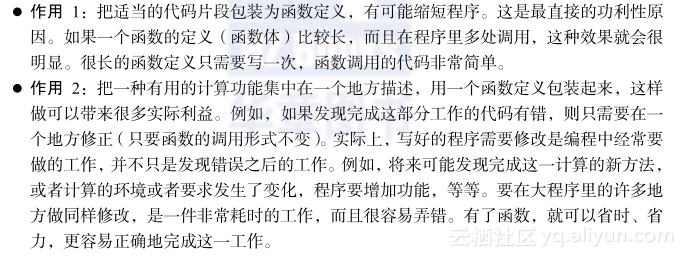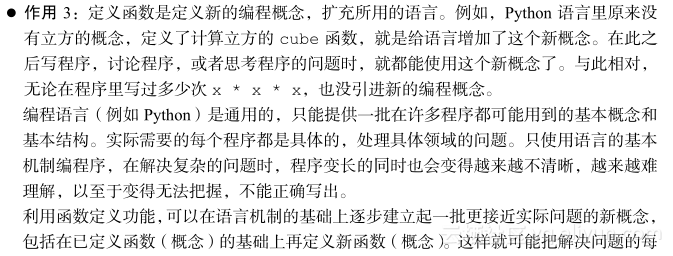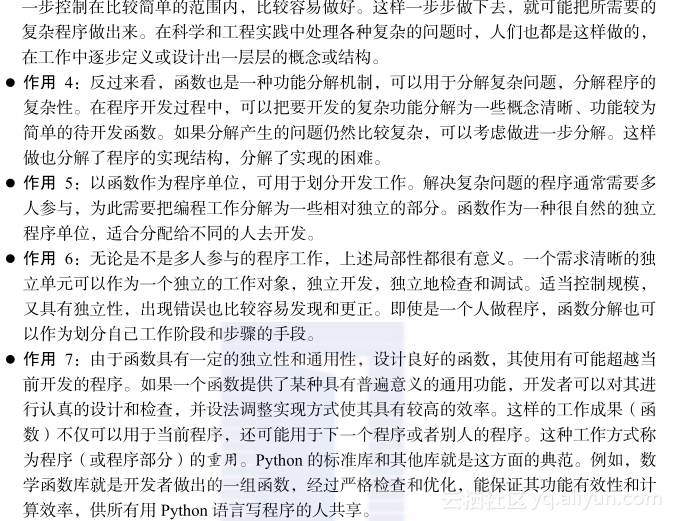函数定义中的工作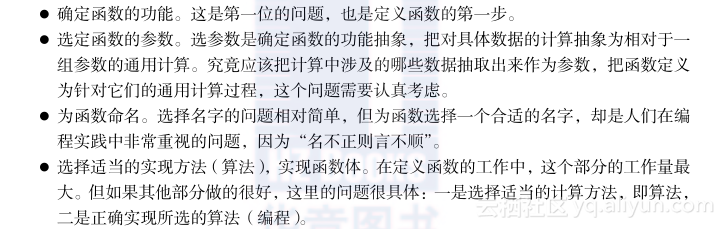程序的函数分解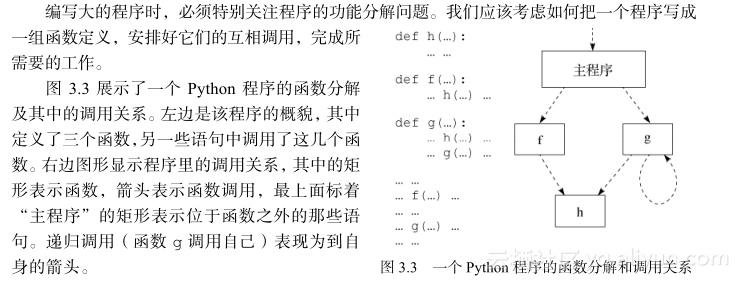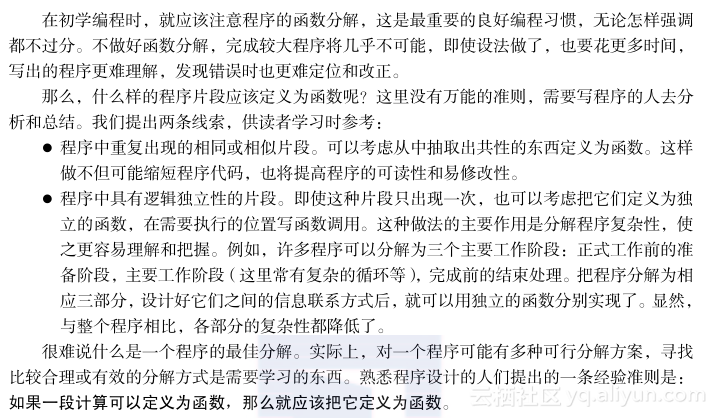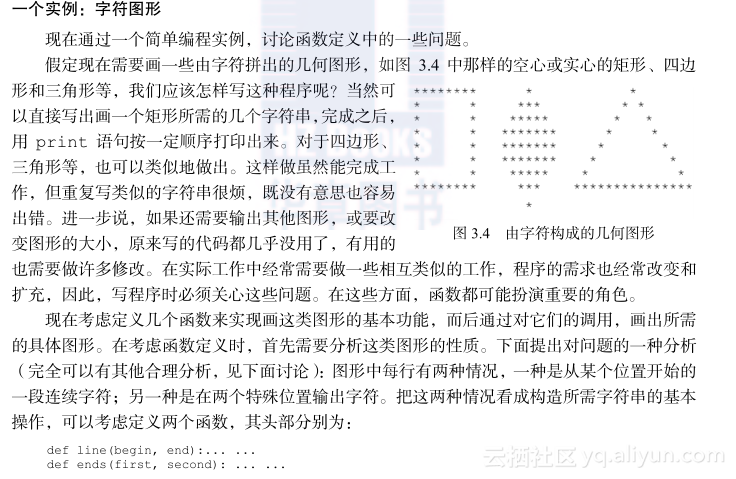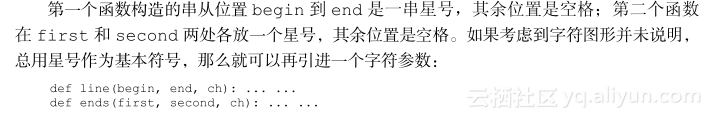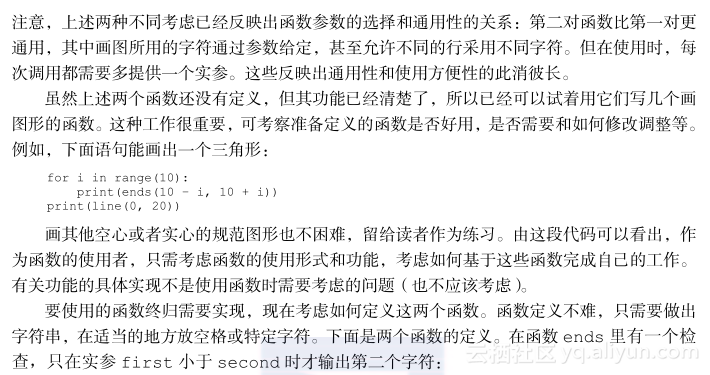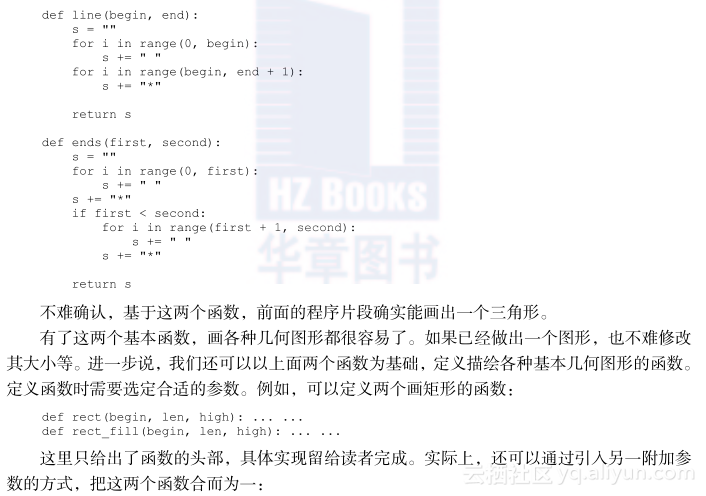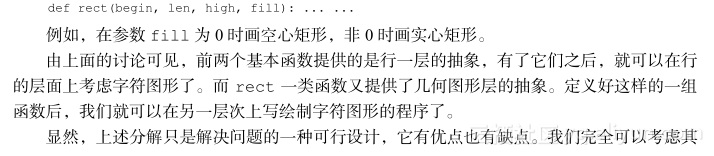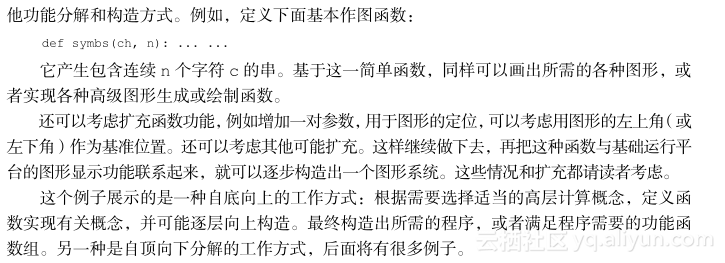函数内部和外部函数的定义def triangle(a, b, c):
if a > 0 and b > 0 and c > 0 and \
a + b > c and b + c > a and a + c > b:
s = (a + b + c)/2
return (s * (s – a) * (s – b) * (s – c))**0.5
else:
return float("nan")

def triangle(a, b, c):
if (ininstance(a, int) or isinstance(a, float)) and \
(ininstance(b, int) or isinstance(b, float)) and \
(ininstance(c, int) or isinstance(c, float)) and \
a > 0 and b > 0 and c > 0 and \
a + b > c and b + c > a and a + c > b:
s = (a + b + c)/2
return (s * (s – a) * (s – b) * (s – c))**0.5
else:
return float("nan")

>>> print(abs.__doc__)
abs(number) -> number

Return the absolute value of the argument.

函数的调用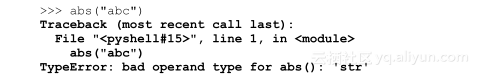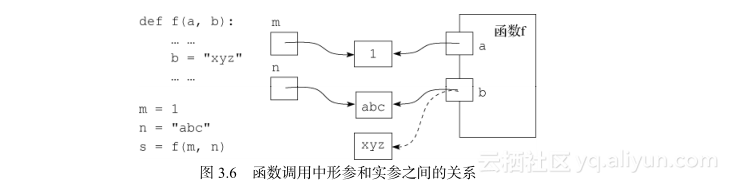函数定义和调用的关系

1）保证只用符合函数实际需要的实参去调用。这就要求在每个函数调用前检查实参的值，满足条件时才调用函数。这件事可以用if语句做。但是这里也有麻烦：没有通过检查的情况怎么办？还是处理错误数据的问题，逃不掉。

2）在调用函数之后检查结果，确定返回值正确后再使用，不正确的情况另行处理。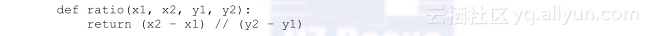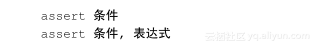def fact(n):
assert isinstance(n, int) and n >= 0
prod = 1
for k in range(2, n+1):
prod = prod * k
return prod

>>> fact(-2)
Traceback (most recent call last):
File "<pyshell#4>", line 1, in <module>
fact(-2)
File "D:/Progs/pyptop-02/fact-assert.py", line 4, in fact
assert n >= 0
AssertionError

def fact(n):
assert isinstance(n, int) and n >= 0, "Argument is " + str(n)
prod = 1
for k in range(2, n+1):
prod = prod * k
return prod

>>> fact(-3)
Traceback (most recent call last):
File "<pyshell#3>", line 1, in <module>
fact(-3)
File "D:\MyBooks\ptop-Python\Progs\basic.py", line 98, in fact
assert isinstance(n, int) and n >= 0, "Argument is " + str(n)
AssertionError: Argument is -3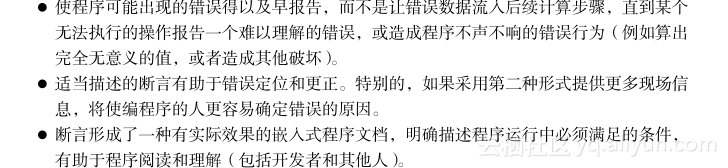通用求解方法

def cbrt(x):
x = float(x)  # 能转换也说明 x 是合理的参数
sign = -1 if x < 0.0 else 1
x = abs(x)
test, root = 0.0, 0.0

while test**3 <= x:
if abs(test**3 - x) < abs(root**3 - x):
root = test
test += 0.0001

return sign * root

>>> cbrt(2)**3
1.9956169789998675
>>> cbrt(20)**3
19.990770343995848
>>> cbrt(200)**3
199.9963601920295
>>> cbrt(2000)**3
1999.899757798265

def cbrt(x):
y = abs(x)
a, b = 0.0, y
while True:
m = (a + b)/2
if abs(m**3 - y) < 0.001:
return -m if x < 0.0 else m
if m**3 > y:
b = m
else:
a = m

>>> cbrt(200.0)**3
199.9990560136106
>>> cbrt(20000.0)**3
20000.000561170757

if y >= 1:
a, b = 1.0, y
else:
a, b = y, 1.0

专用方法def cbrt(x):
if x == 0.0:
return 0.0
x1 = x
while True:
x1 = (2.0 * x1 + x / x1 / x1) / 3
if abs(x1**3 - x) < 0.001:
return x1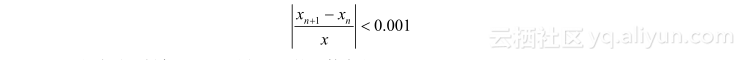def cbrt(x):
if x == 0.0:
return 0.0
x1 = x
while True:
x2 = (2.0 * x1 + x / x1 / x1) / 3
if abs((x2 - x1) / x1) < 0.001:
return x2
x1 = x2

>>> cbrt(200.0)**3
200.00001782197174
>>> cbrt(0.01)**3
0.010000000022781627882 0《从问题到程序：用Python学编程和计算》——2.10　若干Python机制及其他
905 01202 0.NET Core是一个开源通用的开发框架，支持跨平台, 阿里云函数计算推出了 dotnetcore2.1 runtime， 使用 C# 编写 serverless 函数， 除了很好地支持通常意义上的函数外， 还可以基于函数计算开发 asp.
4539 0887 0《从问题到程序：用Python学编程和计算》——2.11　补充材料
1743 0《算法设计编程实验：大学程序设计课程与竞赛训练教材》——3.3　积性函数的实验范例

1212 0《并行计算的编程模型》一3.6.4　wait和wait_until

844 0Java 8：使用compose和andThen组合函数
1021 0776 0
10059

0

+ 订阅

《2021云上架构与运维峰会演讲合集》

《零基础CSS入门教程》

《零基础HTML入门教程》